# MCAT Biology : Covalent Bonds and Hybrid Orbitals

## Example Questions

← Previous 1 3

### Example Question #1 : Covalent Bonds And Hybrid Orbitals

Under normal circumstances, which of the following carbons will be sp3 hybridized?

Carbanion

Carbocation

Carbon involved in a double bond

Carbanion

Explanation:

Carbanions are usually sp3 hybridized. Free radical carbons, carbocations, and double bonded carbons are all sp2 hybridized.

### Example Question #1 : Covalent Bonds And Hybrid Orbitals

Among the most important pH buffer systems in humans is the bicarbonate buffer, which keeps the blood at a remarkably precise 7.42 pH.  The bicarbonate buffer system uses a series of important compounds and enzymes to make the system function.  Figure 1 depicts the key reactions that take place.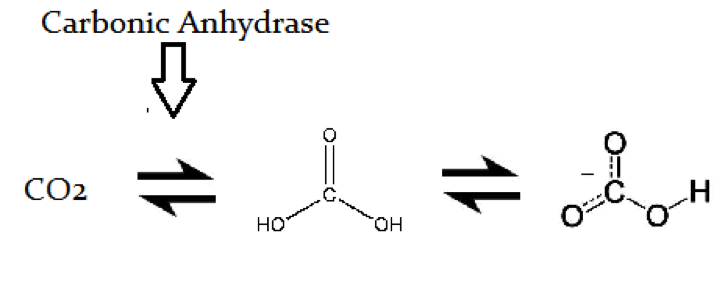The activity of this buffer system is mainly controlled by the renal and respiratory systems.  The renal system excretes bicarbonate in the urine, while the respiratory system “blows off” carbon dioxide as needed.   By balancing these two systems as needed, blood pH is maintained in such a narrow range.

The bond that is present between the carbon atom and the carbonyl oxygen atom in carbonc acid is best described as having which of the following?

One pi bond, one sigma bond

Two sigma bonds

Resonance only when the acid is protonated

Two pi bonds

Two bonds with half pi character and half sigma chacter

One pi bond, one sigma bond

Explanation:

The bond in question is a double bond, and thus is composed of one sigma and one pi bond.

### Example Question #2 : Covalent Bonds And Hybrid Orbitals

How many pi bonds are present in benzylamine?

Seventeen

Three

Four

Eight

Three

Explanation:

Benzylamine consists of a benzene ring with a -CH2NH2 substituent. The benzene ring contains three double bonds, and the substituent has none.

Each bond in a compound represents a sigma bond. Each additional bond represents a pi bond; thus, double binds result from one sigma and one pi bond, and triple bonds from one sigma and two pi bonds. In benzylamine, there are three double bonds and seventeen total bonds, three of which are double bonds. The compound will have a total of seventeen sigma bonds and three pi bonds.

### Example Question #4 : Covalent Bonds And Hybrid Orbitals

Compound has a molecular formula of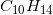. After treatment of compound with hydrogen in the presence of a palladium and a carbon catalyst, a new compound is formed with a molecular formula of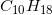. How many rings does compound have?

Zero

Five

One

Two

Four

Two

Explanation:

Recall the formula for degrees of unsaturation as the sum of the number of pi bonds and the number of rings. This value is equivalent to the below formula.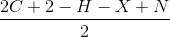C is the number of carbons, H is the number of hydrogens, X is the number of halogens, and N is the number of nitrogens. Using this formula, compound has four degrees of unsaturation, while the product only has two.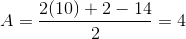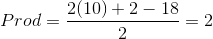The reaction described is a hydrogenation reaction, which will reduce double bonds. The reaction saturates two pi bonds of compound A, meaning the remaining degrees of unsaturation in that compound have to come from two rings. Essentially, due to the reaction given, we know that the product contains no double bonds but still has two degrees of unsaturation. These unsaturation points must correspond to rings.

### Example Question #5 : Covalent Bonds And Hybrid Orbitals

How many bonds are present in this molecule?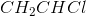Five sigma bonds and two pi bonds

Four sigma bonds and two pi bonds

Five sigma bonds and one pi bond

Four sigma bonds and one pi bond

Three sigma bonds and three pi bonds

Five sigma bonds and one pi bond

Explanation:

The molecule has four single bonds and one double bond.

The first carbon forms two single bonds with the two hydrogen atoms, and a double bond to the second carbon. The second carbon has single bonds with the third hydrogen and the chlorine atom. In total, each carbon has two single bonds and one double bond (to each other), for a total of four single bonds and one double bond.

Each single bond contains one sigma bond and zero pi bonds. Each double bond contains one sigma bond and one pi bond. This molecule has five sigma bonds, and one pi bond.

### Example Question #6 : Covalent Bonds And Hybrid Orbitals

Which of the following compounds has five degrees of unsaturation?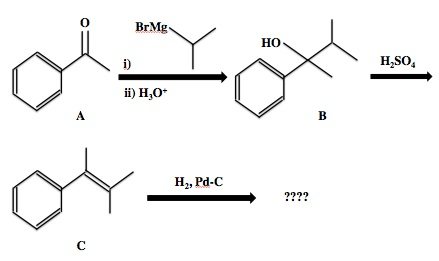Compound C

Compounds A, B, and C

Compounds B and C

Compounds A and C

Compound A

Compounds A and C

Explanation:

A degree of unsaturation results from either a ring or a double bond. In the case of a benzene ring or phenyl substituent, four degrees of unsaturation are present: the ring itself and the three included double bonds.

Compounds A, B, and C all possess a benzene ring, but compounds A and C also have one additional degree of unsaturation. Compound A contains a carbon-oxygen double bond in the ketone group and compound C contains a carbon-carbon double bond in the alkene group. In addition to the four degrees from the ring, these additions bring their total degrees of unsaturation to five. Compound B has no additional double bond, and therefore has four degrees of unsaturation.

### Example Question #1 : Covalent Bonds And Hybrid Orbitals

The degree of unsaturation for ephedrine (shown below) is __________.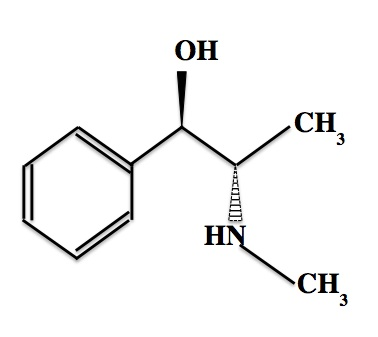Explanation:

The degree of unsaturation is equal to the number of rings plus the number of pi bonds in a molecule. Ephedrine has one ring and three pi bonds, so its degree of unsaturation is four.

To arrive at this answer, one could also use the formula below, whereis the number of carbon atoms,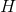is the number of hydrogen atoms,is the number of halogen atoms, andis the number of nitrogen atoms.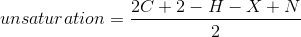For ephedrine,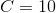,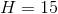,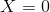, and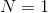.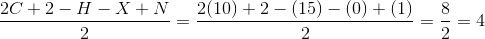### Example Question #1 : Identifying Aromatic Compounds

Compound B.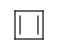Which compound(s) shown above is(are) aromatic?

D

A and C

A, B, and C

A

A

Explanation:

For a compound to be considered aromatic, it must be flat, cyclic, and conjugated and it must obey Huckel's rule. Huckel's rule states that an aromatic compound must have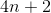pi electrons in the overlapping p orbitals in order to be aromatic (n in this formula represents any integer). Only compounds with 2, 6, 10, 14, . . .  pi electrons can be considered aromatic. Compound A has 6 pi electrons, compound B has 4, and compound C has 8. This eliminates answers B and C. Answer D is not cyclic, and therefore cannot be aromatic. The only aromatic compound is answer choice A, which you should recognize as benzene.

### Example Question #7 : Covalent Bonds And Hybrid Orbitals

How many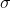-bonds are in one molecule of acetic acid?

Six

Twelve

Three

Seven

Two

Seven

Explanation:

Acetic acid has the formula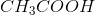. This compound is common enough that the IUPAC name will rarely be given, and you will need to recognize it by common name alone.

Whenever two atoms are directly bonded to one another, a-bond is formed. Even when there is a double or triple bond between two atoms, there is still one-bond between the two atoms. In acetic acid, there are seven bonds between atoms. Even though the carbonyl carbon has a double bond, there is still a-bond between the carbon and oxygen. As a result, there are seven total-bonds in a molecule of acetic acid.

### Example Question #8 : Covalent Bonds And Hybrid Orbitals

Drain cleaners a common household staple, used to open clogged drains in bathtubs and sinks. Human hair is a common culprit that clogs pipes, and hair is made predominately of protein. Drain cleaners are effective at breaking down proteins that have accumulated in plumbing. Drain cleaners can be either acidic or basic, and are also effective at breaking down fats that have accumulated with proteins.

A typical reaction—reaction 1—which would be expected for a drain cleaner on contact with human hair, would be as follows in an aqueous solution: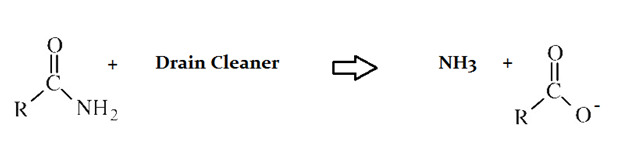Another reaction that may occur, reaction 2, would take place as follows in an aqueous solution: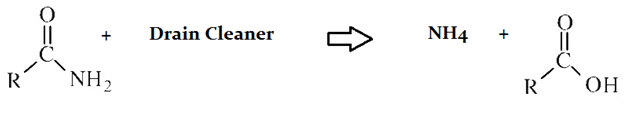In the carbonyl bonds of the preceeding passage __________.

The carbon exhibits sp3 hybridization

The carbon exhibits sp hybridization

Pi bonds result from pure s orbital overlap

Sigma bonds exist above and below the plane of the pi bonds

The carbon exhibits sp2 hybridization